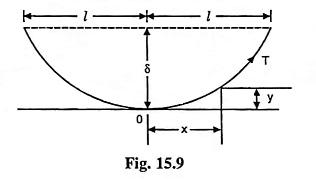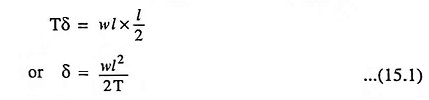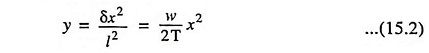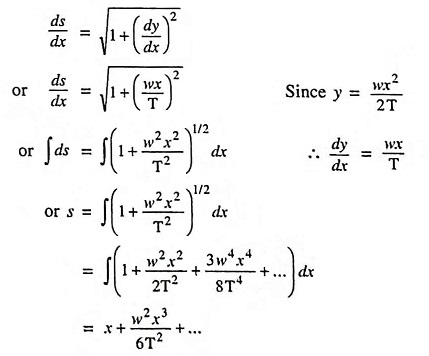## Calculation of Sag and Tension for Trolley Wire in Electric Traction:

Calculation of Sag and Tension for Trolley Wire in Electric Traction – A flexible wire when suspended horizontally between two supports it would assume the form of catenary. But in case of tramways the sag in the trolley wire rarely exceeds 1 or 1 1/2 per cent of the span length and, therefore, the wire may be considered to hang in the form of a parabola.

If 2l is the span length in metres, w is the weight in kg per metre length of wire and T is the tension of wire in kg.Then by taking moments about one of the supports, we getThe equation of parabola is given by the expressionWe know thatWhen x = l i.e., half of the span, the length of conductor in half of the span,

The change in temperature causes a change in length which will cause the change in sag and tension in a span. It should be noted that decrease in temperature reduces the sag and increases the tension. The reduction in the sag causes stretching which on the contrary tends to decrease the sag. In view of this combined effect the elasticity of the conductor material play an important role in determining the exact values of the sag at different temperatures.

Scroll to Top